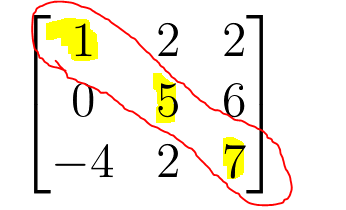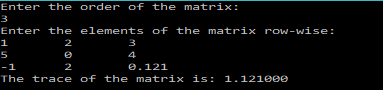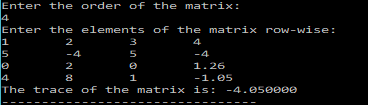# C Program to calculate the TRACE of a Matrix

In the last two posts, we got familiar with 2-D arrays, by writing programs, to add/subtract and multiply matrices.

In this post we will write a C program to find the Trace of a matrix.

If you haven’t read the last two posts, yet then I will recommend you read them before, reading on.
2. Multiply matrices using C

Now, the trace is a mathematical object defined for square matrices.
The trace of a square matrices, is the sum of the diagonal terms.
Let$A$ be an$n\times n$ matrix. Then the trace is given as:$Tr(A) = \sum_{i=1}^{n}(a_{ii})$Using, this info, we can write a simple program that reads a matrix and prints the trace.
In this program I will be using two functions.
One for calculating the trace.
The other for reading the matrix.

### Code:

/******************************************
***********TRACE OF A MATRIX***************
******************************************/
#include<stdio.h>
/********
Function that calculates and returns the trace of a square matrix
********/
double trace(int n,double a[n][n]){
int i;
double sumDiag=0;
for(i=0;i<n;i++){
sumDiag=sumDiag+a[i][i];
}
return sumDiag;
}
/*******
Function that reads the elements of a matrix row-wise
Parameters: rows(m),columns(n),matrix[m][n]
*******/
void readMatrix(int m, int n, double matrix[m][n]){
int i,j;
for(i=0;i<m;i++){
for(j=0;j<n;j++){
scanf("%lf",&matrix[i][j]);
}
}
}
main(){
double tr;
int n,i,j;
printf("Enter the order of the matrix:\n");
scanf("%d",&n);
double a[n][n];
printf("Enter the elements of the matrix row-wise:\n");
tr=trace(n,a);
printf("The trace of the matrix is: %lf",tr);

}


The program is pretty much self-explanatory. I ask the user to enter the size(order) of the matrix. Then, I declare a matrix(2-d array) of the given size. Then we prompt the user to enter the elements of the matrix, and read them by calling the function in the beginning. Finally, the trace is calculated by calling the trace function.

### OUTPUT:

A sample run of the program is shown below: